Gradient, slope, and aspect of data grid

## Syntax

``[aspect,slope,gradN,gradE] = gradientm(F,R)``
``[aspect,slope,gradN,gradE] = gradientm(F,R,spheroid)``
``[aspect,slope,gradN,gradE] = gradientm(lat,lon,F)``
``[aspect,slope,gradN,gradE] = gradientm(lat,lon,F,spheroid)``
``[aspect,slope,gradN,gradE] = gradientm(lat,lon,F,spheroid,angleUnit)``

## Description

### Regular Data Grids

example

````[aspect,slope,gradN,gradE] = gradientm(F,R)` returns the aspect angle, slope angle, and north and east components of the gradient for a regular data grid `F` with respect to a geographic reference `R`. By default, `gradientm` locates the latitude and longitude coordinates referenced by `R` using the spheroid contained in the `Spheroid` property of the `geocrs` object in the `GeographicCRS` property of `R`. If the `GeographicCRS` property of `R` is empty, then `geopeaks` uses GRS80.```
````[aspect,slope,gradN,gradE] = gradientm(F,R,spheroid)` uses the specified reference spheroid instead of the spheroid contained in the `Spheroid` property of the `geocrs` object in the `GeographicCRS` property of `R` or the spheroid GRS80.```

### Geolocated Data Grids

````[aspect,slope,gradN,gradE] = gradientm(lat,lon,F)` returns the same values for a geolocated data grid `F` with respect to the latitude-longitude mesh defined by `lat` and `lon`. By default, latitude and longitude are in degrees. The default reference spheroid is GRS80.```
````[aspect,slope,gradN,gradE] = gradientm(lat,lon,F,spheroid)` uses the specified reference spheroid instead of GRS80.```
````[aspect,slope,gradN,gradE] = gradientm(lat,lon,F,spheroid,angleUnit)` specifies the units for latitude and longitude as `'degrees'` (the default) or `'radians'`.```

## Examples

collapse all

Generate sample elevation data for the region around a mountain summit using a geographic postings reference object and the `geopeaks` function. To do this, first create a reference object for the region by specifying the latitude and longitude limits and the size of the elevation data grid. Next, generate elevation data for the region using `geopeaks`.

```latlim = [10 45]; lonlim = [60 100]; size = [100 100]; R = georefpostings(latlim,lonlim,size); F = geopeaks(R);```

Compute the aspect angles, slope angles, and gradient components of the data.

`[aspect,slope,gradN,gradE] = gradientm(F,R);`

Visualize the results by plotting the data. First, plot the elevation data using an equidistant cylindrical projection. To do this, create a set of map axes and specify the projection using `axesm`. Plot the data as a surface using `geoshow`, and adjust the aspect ratio of the axes using `daspect`. Display a 3-D view of the axes using `view`.

```figure axesm('eqdcylin'); geoshow(F,R,'DisplayType','surface') daspect([1 1 5]) title('Elevation Data') colorbar view(3)```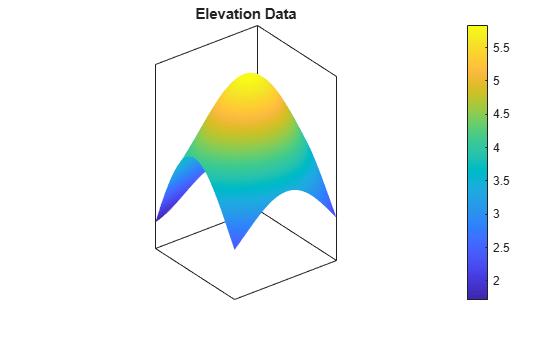Then, plot the gradient components using the same projection. Note that both the north and east component values are zero at the summit.

```figure axesm('eqdcylin') geoshow(gradN,R,'DisplayType','surface') title('North Components of Gradient') colorbar```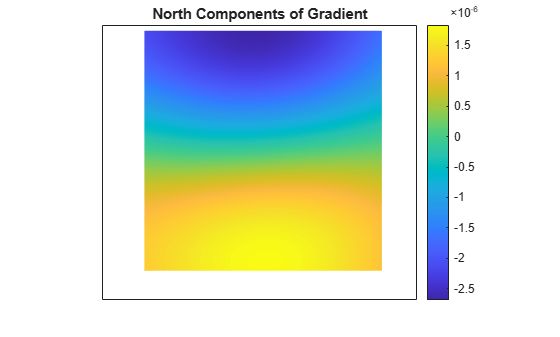```figure axesm('eqdcylin') geoshow(gradE,R,'DisplayType','surface') title('East Components of Gradient') colorbar```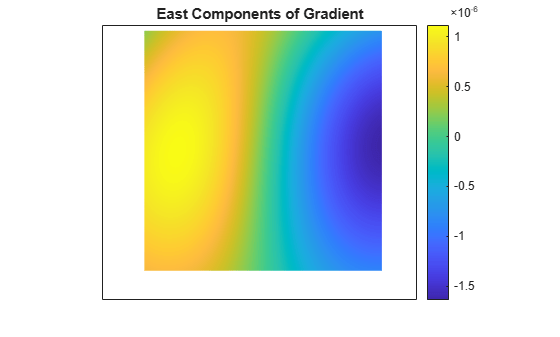Plot the slope angles. Note that the value of the slope angle is zero at the summit.

```figure axesm('eqdcylin') geoshow(slope,R,'DisplayType','surface') title('Slope Angles') colorbar```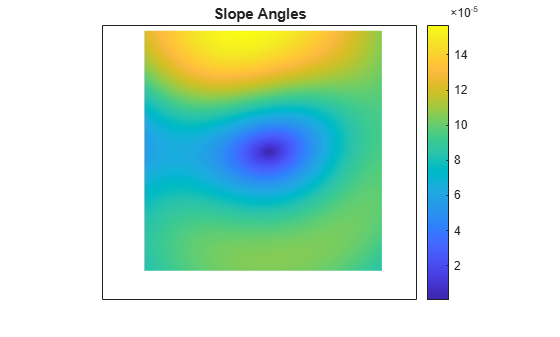Plot the aspect angles. An aspect angle describes the direction the mountain slope faces as an azimuth measured clockwise from north.

```figure axesm('eqdcylin') geoshow(aspect,R,'DisplayType','surface') title('Aspect Angles') colorbar```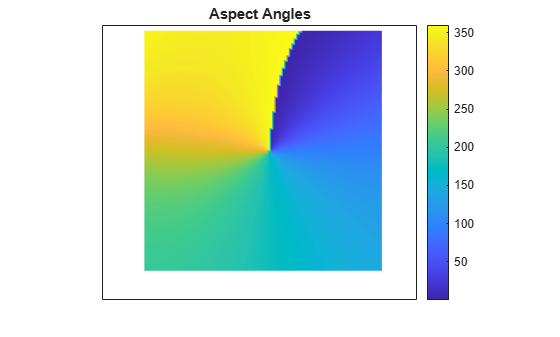## Input Arguments

collapse all

Data grid, specified as a numeric matrix with at least two rows and two columns. The data grid may contain `NaN` values. `F` is either a regular data grid associated with a geographic raster reference object, or a georeferenced data grid with respect to a latitude-longitude mesh.

If `F` is a regular data grid and `R` is a reference object, then `size(F)` must be the same as `R.RasterSize`. If `F` is a geolocated data grid, then `size(F)` must be the same as `size(lat)` and `size(lon)`.

Data Types: `single` | `double`

Geographic reference that contains geospatial referencing information for `F`, specified as one of these values:

• `GeographicCellsReference` or `GeographicPostingsReference` object, where `R.RasterSize` is the same as `size(F)`.

• 3-by-2 numeric matrix that associates the row and column indices of a data grid with geographic coordinates, such that ```[lon lat] = [row col 1] * R```. The matrix must define a nonrotational and nonskewed relationship in which each column of the data grid falls along a meridian and each row falls along a parallel.

• 1-by-3 numeric vector with elements `[s nlat wlon]`, where `s` is the number of data grid samples per degree, `nlat` is the northernmost latitude of the data grid in degrees, and `wlon` is the westernmost longitude in degrees.

Reference spheroid, specified as a `referenceEllipsoid` object, `oblateSpheroid` object, `referenceSphere` object, or vector of the form ```[semimajorAxis eccentricity]```.

Example: ```spheroid = referenceEllipsoid(wgs84Ellipsoid);```

Latitudes, specified as a numeric matrix with at least two rows and two columns. By default, specify latitudes in degrees. To use values in radians, specify the `angleUnit` argument as `'radians'`.

`lat` must be the same size as `lon` and `F`.

Data Types: `single` | `double`

Longitudes, specified as a numeric matrix with at least two rows and two columns. By default, specify longitudes in degrees. To use values in radians, specify the `angleUnit` argument as `'radians'`.

`lon` must be the same size as `lat` and `F`.

Data Types: `single` | `double`

Angle units, specified as `'degrees'` (the default) or `'radians'`.

## Output Arguments

collapse all

Aspect angles, returned as a matrix of the same size as `F`. An aspect angle is the direction in which `F` decreases most rapidly, expressed as an azimuth measured clockwise from north.

By default, aspect angles are in degrees. To return values in radians, specify `lat` and `lon` in radians and `angleUnit` as `'radians'`.

If both components of the gradient are zero, then the aspect angle is returned as `NaN`.

Data Types: `double`

Slope angles, returned as a matrix of the same size as `F`. For the slope angles to have physical meaning, the data grid must specify elevation, and its distance unit must match the length unit of the reference spheroid. Otherwise, a slope angle is the arctangent of the magnitude of the gradient.

By default, slope angles are in degrees. To return values in radians, specify `lat` and `lon` in radians and `angleUnit` as `'radians'`.

Data Types: `double`

North components of the gradient, returned as a matrix of the same size as `F`. The north component of a gradient is the change in `R` per unit of distance in the north direction, where the distance unit matches the length unit of the reference spheroid.

Data Types: `double`

East components of the gradient, returned as a matrix of the same size as `F`. The east component of a gradient is the change in `R` per unit of distance in the east direction, where the distance unit matches the length unit of the reference spheroid.

Data Types: `double`

## Version History

Introduced before R2006a